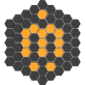# Set point by 3point pick and link selected objects to point

Hello everyone,
is ther a way to create a point from a 3point pick and link the selcted ojbets to it.
F.e. if you has a Lamp and you want to set a Masterpoint to the top.

step1: on selected objects
step2: 3point pick create point
step3: linked all selected objects to point

See attachement

Thx

AttachmentSize

## Comment viewing options### .

```(
if selection.count != 0 do
(
local pos01 = undefined
local pos02 = undefined
local pos03 = undefined

local curSnapMode = snapMode.active
local curSnapType = snapMode.type
snapMode.active = true
snapMode.type = #3D

pos01 = pickPoint snap:#3d
if classof pos01 == point3 do
pos02 = pickPoint snap:#3d rubberBand:pos01
if classof pos02 == point3 do
pos03 = pickPoint snap:#3d rubberBand:pos02

snapMode.active = curSnapMode
try(snapMode.type = curSnapType)catch()
if classof pos01 == point3 and classof pos02 == point3 and classof pos03 == point3 do
(
p = point pos:((pos01 + pos02 + pos03) / 3.0)
selection.parent = p
)
)
)```### Thx, you are the hero we

Thx,
you are the hero we beginners all need,
just one question what if the 3 point selection defines a circle and the point should been set to the middle of the circle and not like now where its set to the middle of the triangle.### .

Since the script can't know where you wnat the point to be placed use the code above when you want the point to be at the center of the triangle formed by the picked points and use the code below when you want the point to be placed at the center of the circle formed by the picked points.

```(
fn barycentricToWorld pos1 pos2 pos3 u v w =
(u * pos1 + v * pos2 + w * pos3) / (u + v + w)
fn getCircumcircle pos1 pos2 pos3 =
(
local a = pos3 - pos2
local b = pos3 - pos1
local c = pos2 - pos1

local u = dot a a * dot c b
local v = dot b b * dot c -a
local w = dot c c * dot b a

local center = barycentricToWorld pos1 pos2 pos3 u v w
)
--

if selection.count != 0 do
(
local pos01 = undefined
local pos02 = undefined
local pos03 = undefined

local curSnapMode = snapMode.active
local curSnapType = snapMode.type
snapMode.active = true
snapMode.type = #3D

pos01 = pickPoint snap:#3d
if classof pos01 == point3 do
pos02 = pickPoint snap:#3d rubberBand:pos01
if classof pos02 == point3 do
pos03 = pickPoint snap:#3d rubberBand:pos02

snapMode.active = curSnapMode
try(snapMode.type = curSnapType)catch()
if classof pos01 == point3 and classof pos02 == point3 and classof pos03 == point3 do
(
p = point pos:(getCircumcircle pos01 pos02 pos03)
selection.parent = p
)
)

)```